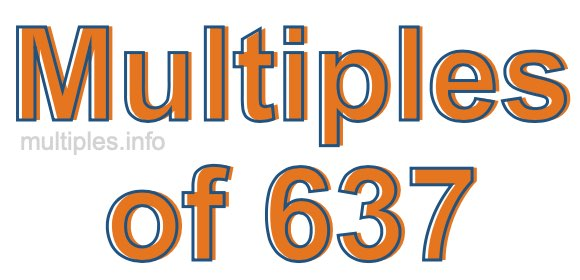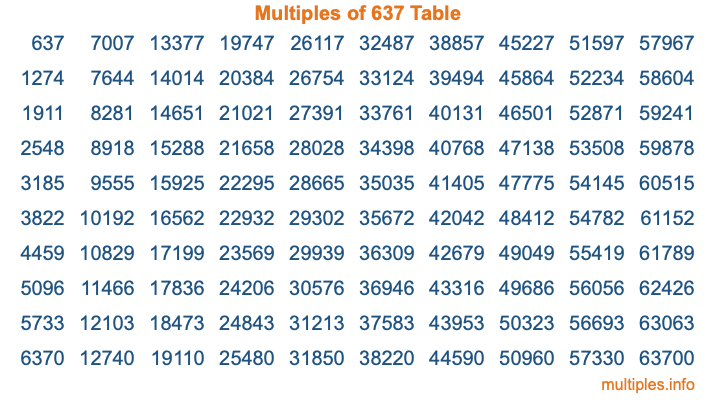Multiples of 637Welcome to the Multiples of 637 page. Here we will first teach you everything you will ever need to know about the multiples of 637, and then give you a study guide summary of everything we taught you to make sure you remember it all. Use this page to look up facts and learn information about the multiples of 637. This page will make you a multiples of six hundred thirty-seven expert!

Definition of Multiples of 637
Multiples of 637 are all the numbers that when divided by 637 equal an integer. Each of the multiples of 637 are called a multiple. A multiple of 637 is created by multiplying 637 by an integer.

Therefore, to create a list of multiples of 637, you start with 1 multiplied by 637, then 2 multiplied by 637, then 3 multiplied by 637, and so on for as long as you want. Thus, the list of the first five multiples of 637 is 637, 1274, 1911, 2548, and 3185. To see a larger list of multiples of 637, see the printable image of Multiples of 637 further down on this page. We also have a category where you can choose any nth multiple of 637.

Multiples of 637 Checker
The Multiples of 637 Checker below checks to see if any number of your choice is a multiple of 637. In other words, it checks to see if there is any number (integer) that when multiplied by 637 will equal your number. To do that, we divide your number by 637. If the the quotient is an integer, then your number is a multiple of 637.

Is  a multiple of 637?

Least Common Multiple of 637 and ...
A Least Common Multiple (LCM) is the lowest multiple that two or more numbers have in common. This is also called the smallest common multiple or lowest common multiple and is useful to know when you are adding our subtracting fractions. Enter one or more numbers below (637 is already entered) to find the LCM.

Check out our LCM Calculator if you need more details about the Least Common Multiple or if you need the LCM for different numbers for adding and subtraction fractions.

nth Multiple of 637
As we stated above, 637 is the first multiple of 637, 1274 is the second multiple of 637, 1911 is the third multiple of 637, and so on. Enter a number below to find the nth multiple of 637.

th multiple of 637

Multiples of 637 vs Factors of 637
637 is a multiple of 637 and a factor of 637, but that is where the similarities end. All postive multiples of 637 are 637 or greater than 637. All positive factors of 637 are 637 or less than 637.

Below is the beginning list of multiples of 637 and the factors of 637 so you can compare:

Multiples of 637: 637, 1274, 1911, 2548, 3185, etc.

Factors of 637: 1, 7, 13, 49, 91, 637

As you can see, the multiples of 637 are all the numbers that you can divide by 637 to get a whole number. The factors of 637, on the other hand, are all the whole numbers that you can multiply by another whole number to get 637.

It's also interesting to note that if a number (x) is a factor of 637, then 637 will also be a multiple of that number (x).

Multiples of 637 vs Divisors of 637
The divisors of 637 are all the integers that 637 can be divided by evenly. Below is a list of the divisors of 637.

Divisors of 637: 1, 7, 13, 49, 91, 637

The interesting thing to note here is that if you take any multiple of 637 and divide it by a divisor of 637, you will see that the quotient is an integer.

Multiples of 637 Table
Below is an image of the first 100 multiples of 637 in a table. The table is in chronological order, column by column. The first column has the first ten multiples of 637, the second column has the next ten multiples of 637, and so on.The Multiples of 637 Table is also referred to as the 637 Times Table or Times Table of 637. You are welcome to print out our table for your studies.

Negative Multiples of 637
Although not often discussed or needed in math, it is worth mentioning that you can make a list of negative multiples of 637 by multiplying 637 by -1, then by -2, then by -3, and so on, to get the following list of negative multiples of 637:

-637, -1274, -1911, -2548, -3185, etc.

Multiples of 637 Summary
Below is a summary of important Multiples of 637 facts that we have discussed on this page. To retain the knowledge on this page, we recommend that you read through the summary and explain to yourself or a study partner why they hold true.

There are an infinite number of multiples of 637.

A multiple of 637 divided by 637 will equal a whole number.

637 divided by a factor of 637 equals a divisor of 637.

The nth multiple of 637 is n times 637.

The largest factor of 637 is equal to the first positive multiple of 637.

637 is a multiple of every factor of 637.

637 is a multiple of 637.

A multiple of 637 divided by a divisor of 637 equals an integer.

637 divided by a divisor of 637 equals a factor of 637.

Any integer times 637 will equal a multiple of 637.

Multiples of a Number
Here you can get the multiples of another number, all with the same attention to detail as we did for multiples of 637 on this page.

Multiples of
Multiples of 638
Did you find our page about multiples of six hundred thirty-seven educational? Do you want more knowledge? Check out the multiples of the next number on our list!